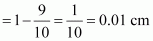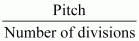# 2.6 Which of the following is the most precise device for measuring length : (a) a vernier callipers with 20 divisions on the sliding scale (b) a screw gauge of pitch 1 mm and 100 divisions on the circular scale (c) an optical instrument that can measure length to within a wavelength of light ?

##### 2.6 Which of the following is the most precise device for measuring length : (a) a vernier callipers with 20 divisions on the sliding scale (b) a screw gauge of pitch 1 mm and 100 divisions on the circular scale (c) an optical instrument that can measure length to within a wavelength of light ?

8 years ago

A device with minimum count is the most suitable to measure length.

(a) Least count of vernier callipers

= 1 standard division (SD) – 1 vernier division (VD)(b) Least count of screw gauge =(c) Least count of an optical device = Wavelength of light ∼ 10–5 cm

= 0.00001 cm

Hence, it can be inferred that an optical instrument is the most suitable device to measure length.